# LeetCode 初級演算法之連結串列，看看你都學會了嗎？

theme: juejin highlight: xcode

# 題一：刪除連結串列中的節點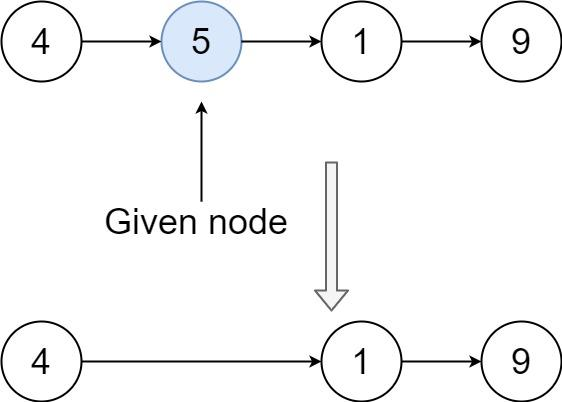```輸入：head = [4,5,1,9], node = 5 輸出：[4,1,9] 解釋：指定連結串列中值為 5 的第二個節點，那麼在呼叫了你的函式之後，該連結串列應變為 4 -> 1 -> 9```

## 解題思路：直接替換

```swift func deleteNode(_ node: ListNode?, _ head: ListNode?) { node?.val = node?.next?.val ?? 0 node?.next = node?.next?.next ?? nil let head = head }```

# 題二：刪除連結串列的倒數第N個節點

## 解題思路：遞迴、雙迴圈、快慢指標

### 遞迴

```swift func removeNthFromEnd(_ head: ListNode?, _ n: Int) -> ListNode? { let pos = length(head, n) if pos == n { return head?.next } return head }

func length(_ node: ListNode?, _ n: Int) -> Int { if node?.next == nil { return 1 } /// 獲取前面一個節點 let pos = length(node?.next, n) + 1 if pos == n+1 { print("(pos)") node?.next = node?.next?.next } return pos } ```

### 雙迴圈

```swift func removeNthFromEnd(_ head: ListNode?, _ n: Int) -> ListNode? { let pos = length(head, n) if pos == n { return head?.next } return head }

func length(_ node: ListNode?, _ n: Int) -> Int { if node?.next == nil { return 1 } /// 獲取前面一個節點 let pos = length(node?.next, n) + 1 if pos == n+1 { print("(pos)") node?.next = node?.next?.next } return pos } ```

### 快慢指標

`fast``slow`兩個指標，`fast`先走`n`次，然後和`slow`一起移動。 `slow``fast`相距`n`， 當`fast`先走到連結串列底部，`slow`就是要刪除的物件；

```swift func removeNthFromEnd3(_ head: ListNode?, _ n: Int) -> ListNode? { var fast = head var slow = head var index = n /// jNode 移位 n while index > 0 { fast = fast?.next index-=1 let j = fast } if fast?.next == nil { return head?.next } /// 開始遍歷 while fast?.next != nil { fast = fast?.next slow = slow?.next } slow?.next = slow?.next?.next return head }```

# 題三：反轉連結串列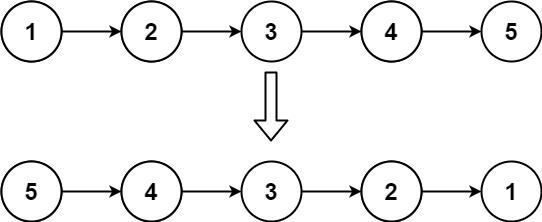```輸入： head = [1,2,3,4,5] 輸出： [5,4,3,2,1]```

## 解題思路：棧、遞迴、雙指標

### 棧操作

```swift func reverseList(_ head: ListNode?) -> ListNode? { /// 全部入棧 var node = head var stack = Stack<ListNode>() while node != nil { if let node = node { stack.push(element: node) } node = node?.next } /// 第一個出棧的節點是新的表頭 node = stack.pop() /// 新的表頭 var newHead = node while !stack.isEmpty() { let tempNode = stack.pop() node?.next = tempNode node = tempNode } node?.next = nil return newHead }```

### 雙指標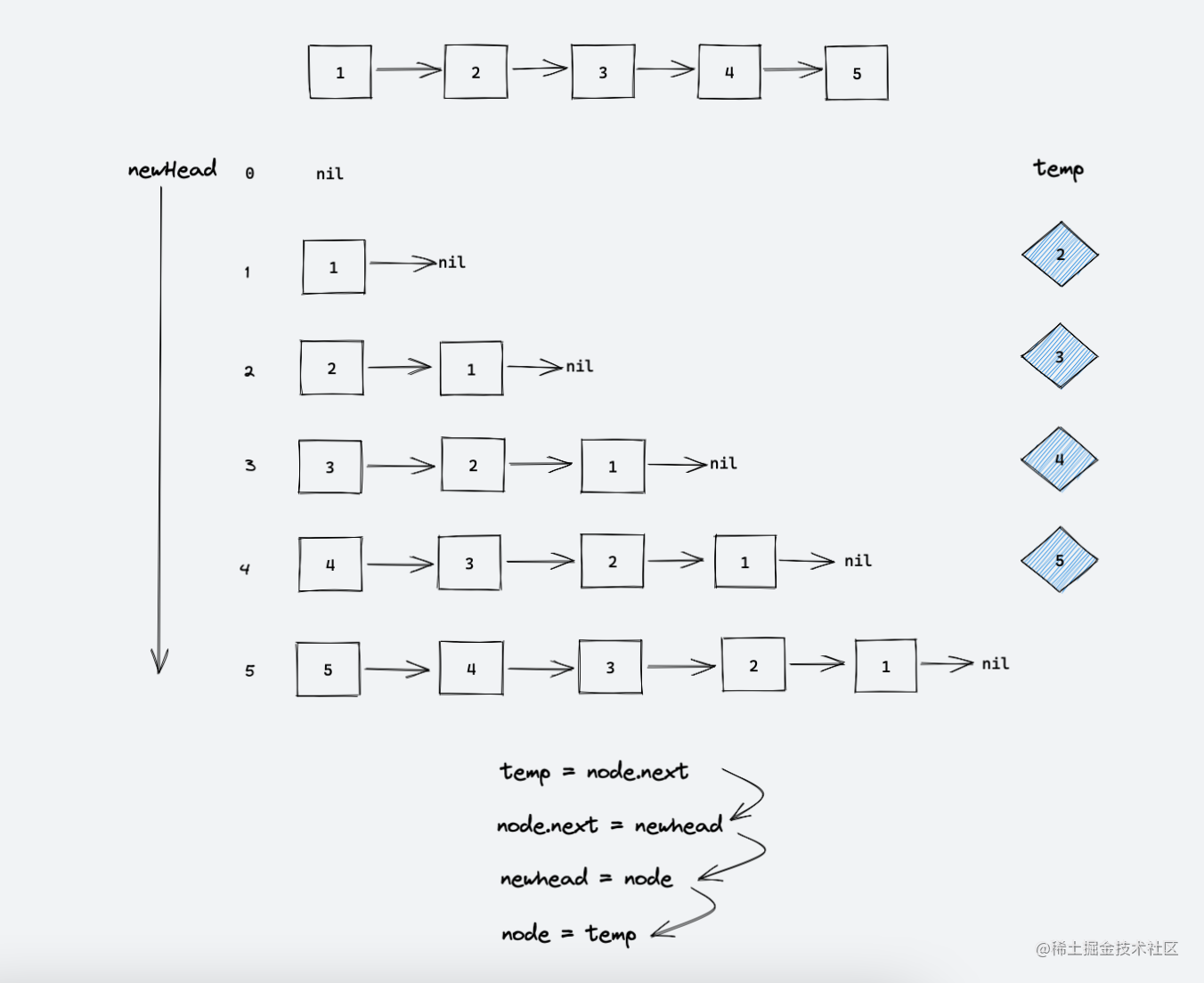```swift func reverseList(_ head: ListNode?) -> ListNode? { var node = head var newhead: ListNode? = nil while node != nil { let temp = node?.next node?.next = newhead newhead = node node = temp } return newhead }```

# 題四：合併兩個有序連結串列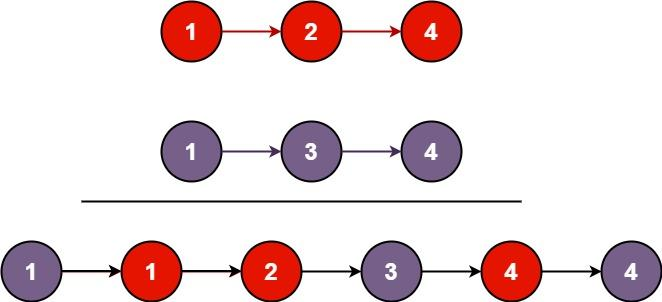```輸入： l1 = [1,2,4], l2 = [1,3,4] 輸出： [1,1,2,3,4,4]```

## 解題思路：雙指標

```swift func mergeTwoLists(_ list1: ListNode?, _ list2: ListNode?) -> ListNode? { var node1 = list1 var node2 = list2 var newHead = ListNode(0) var cur: ListNode? = newHead while node1 != nil && node2 != nil { let val1 = node1?.val ?? 0 let val2 = node2?.val ?? 0 if val1 <= val2 { cur?.next = node1 node1 = node1?.next } else { cur?.next = node2 node2 = node2?.next } cur = cur?.next } cur?.next = node1 == nil ? node2 : node1 let head = newHead.next return head }```

# 題五：迴文連結串列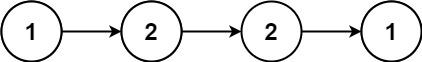```輸入： head = [1,2,2,1] 輸出： true```

## 解題思路：棧操作、遞迴、反轉後半連結串列

### 棧操作

```swift func isPalindrome(_ head: ListNode?) -> Bool { var node = head var stack = Stack<Int>() var length = 0 while node != nil { if let node = node { stack.push(element: node.val) } node = node?.next length+=1 } node = head length/=2 while length > 0 { if node?.val != stack.pop() { return false } node = node?.next length-=1 } return true }```

### 反轉後半連結串列

```swift func isPalindrome(_ head: ListNode?) -> Bool { var fast = head var slow = head while fast != nil, fast?.next != nil { fast = fast?.next?.next slow = slow?.next } /// 奇數就選下一個 if fast != nil { slow = slow?.next } var newNode = reverse(slow) var node = head while newNode != nil { if newNode?.val != node?.val { return false } newNode = newNode?.next node = node?.next } return true }```

# 題六：環形連結串列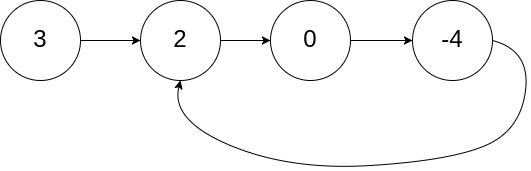```輸入： head = [3,2,0,-4], pos = 1 輸出： true 解釋： 連結串列中有一個環，其尾部連線到第二個節點。``` 示例 2：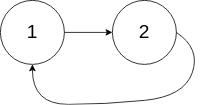```輸入： head = , pos = -1 輸出： false 解釋： 連結串列中沒有環。```

## 解題思路：集合法、快慢指標

### 集合法

```swift func hasCycle(_ head: ListNode?) -> Bool { var node = head?.next var set = Set<ListNode>() while node != nil { if set.contains(node!) { return true } else { set.insert(node!) } node = node?.next } return false }```